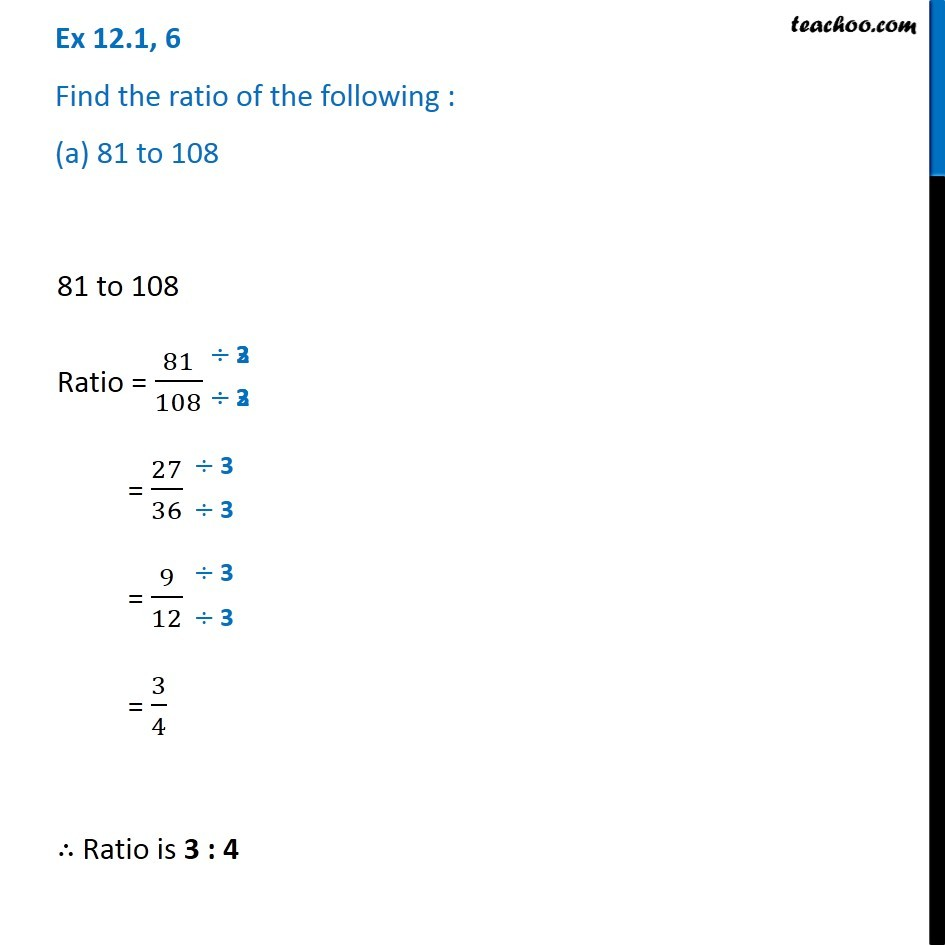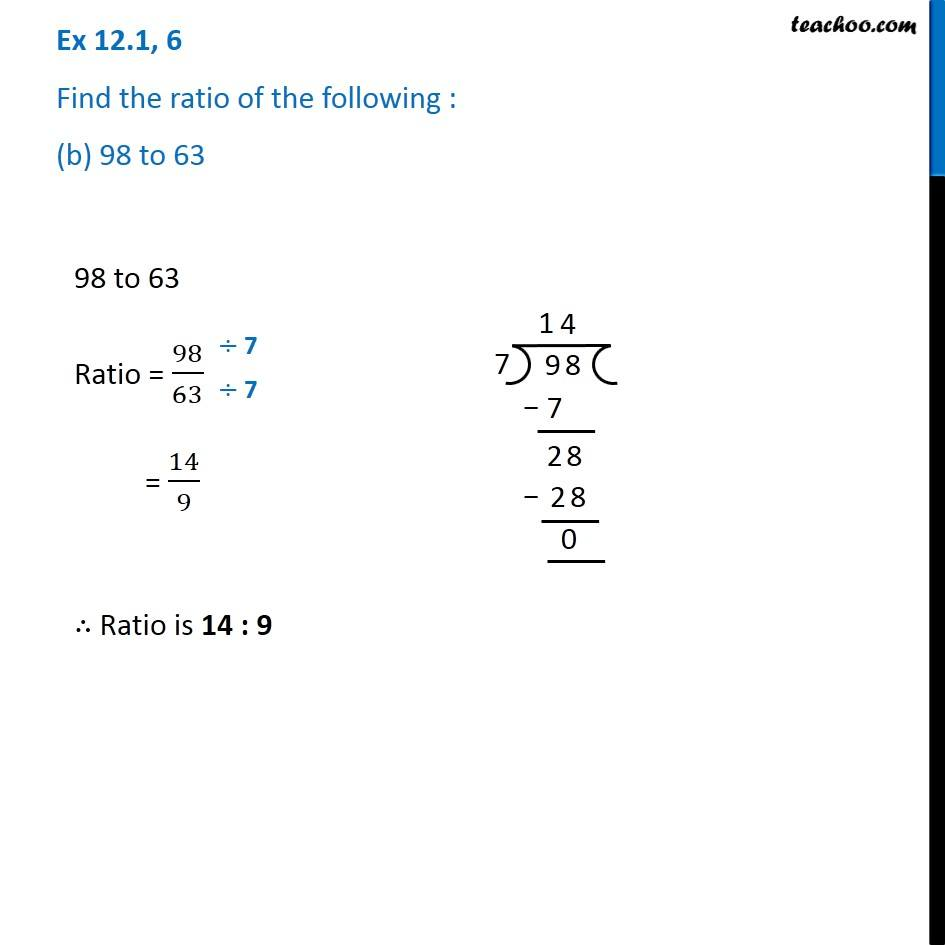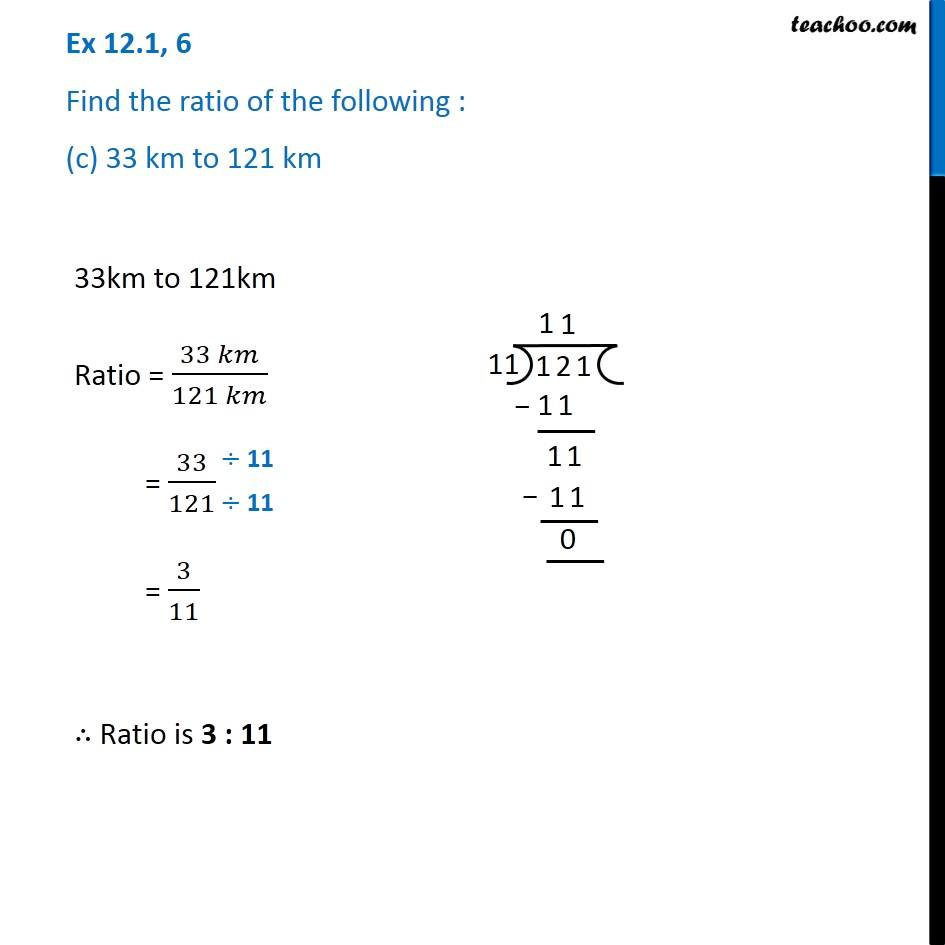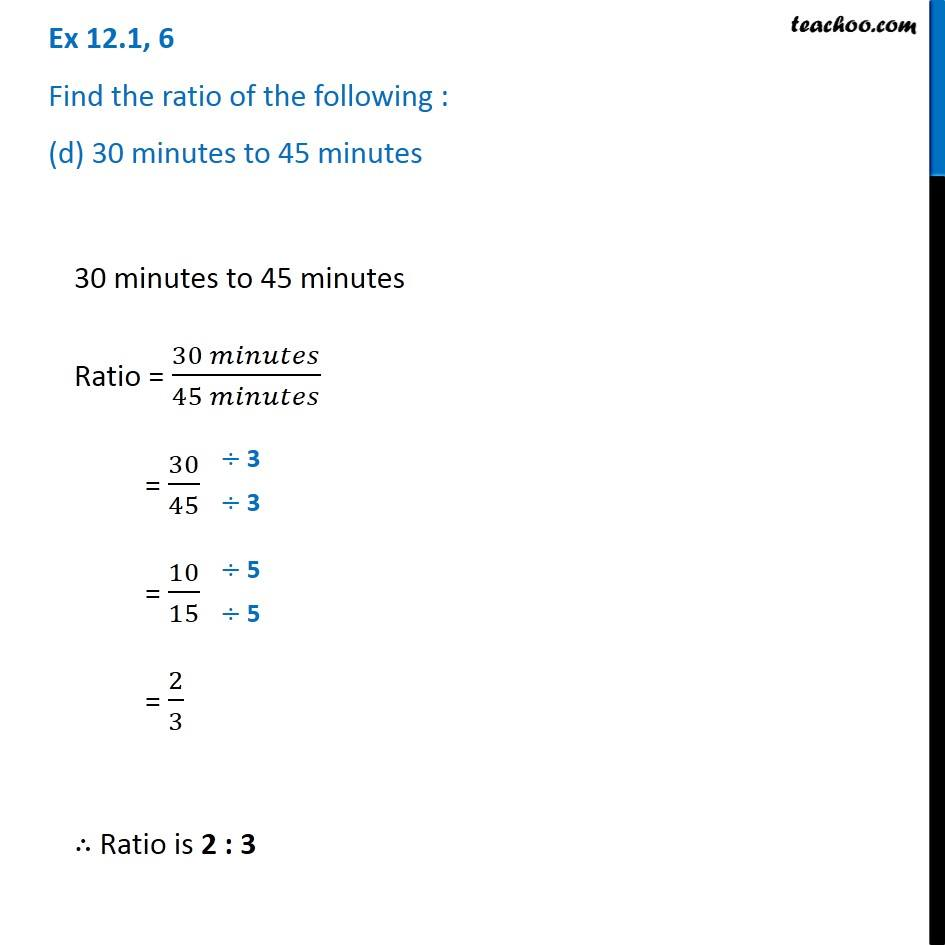1. Chapter 12 Class 6 Ratio And Proportion
2. Concept wise
3. Ratios

Transcript

Ex 12.1, 6 Find the ratio of the following : (a) 81 to 108 81 to 108 Ratio = 81/108 = 27/36 = 9/12 = 3/4 ∴ Ratio is 3 : 4 Ex 12.1, 6 Find the ratio of the following : (b) 98 to 63 98 to 63 Ratio = 98/63 = 14/9 ∴ Ratio is 14 : 9 Ex 12.1, 6 Find the ratio of the following : (c) 33 km to 121 km 33km to 121km Ratio = (33 𝑘𝑚)/(121 𝑘𝑚) = 33/121 = 3/11 ∴ Ratio is 3 : 11 Ex 12.1, 6 Find the ratio of the following : (d) 30 minutes to 45 minutes30 minutes to 45 minutes Ratio = (30 𝑚𝑖𝑛𝑢𝑡𝑒𝑠)/(45 𝑚𝑖𝑛𝑢𝑡𝑒𝑠) = 30/45 = 10/15 = 2/3 ∴ Ratio is 2 : 3

Ratios